NEET  >  Test: Physical World - 1

# Test: Physical World - 1

Test Description

## 10 Questions MCQ Test Physics Class 11 | Test: Physical World - 1

Test: Physical World - 1 for NEET 2023 is part of Physics Class 11 preparation. The Test: Physical World - 1 questions and answers have been prepared according to the NEET exam syllabus.The Test: Physical World - 1 MCQs are made for NEET 2023 Exam. Find important definitions, questions, notes, meanings, examples, exercises, MCQs and online tests for Test: Physical World - 1 below.
Solutions of Test: Physical World - 1 questions in English are available as part of our Physics Class 11 for NEET & Test: Physical World - 1 solutions in Hindi for Physics Class 11 course. Download more important topics, notes, lectures and mock test series for NEET Exam by signing up for free. Attempt Test: Physical World - 1 | 10 questions in 10 minutes | Mock test for NEET preparation | Free important questions MCQ to study Physics Class 11 for NEET Exam | Download free PDF with solutions
 1 Crore+ students have signed up on EduRev. Have you?
Test: Physical World - 1 - Question 1

Detailed Solution for Test: Physical World - 1 - Question 1
• The radioactivity of uranium was discovered in 1896 by Henri Becquerel.
• Though it was Henri Becquerel that discovered radioactivity, it was Marie Curie who coined the term.

Additional Information: Marie Curie became the first woman to be awarded the Nobel prize and the first person to obtain two Nobel prizes when she won the prize for the discovery of Polonium and Radium in 1911.

Test: Physical World - 1 - Question 2

### Which is the strongest fundamental force of nature?

Detailed Solution for Test: Physical World - 1 - Question 2

Among all these 4 forces the Strong nuclear force also called strong nuclear interaction is the strongest one in comparison of the other three.

• It is because it binds the fundamental particles of any matter together to form larger particles.
• Fundamental particles mean protons and neutrons.
• Quarks are made up of protons and neutrons and this strong nuclear force holds together the quarks.
• The strong nuclear force also binds the proton and neutrons to the nucleus of the atom.
• Also, a small strong force acts between protons and neutrons.
• As protons repel each other due to having the same charges, the strong forces also keep them together.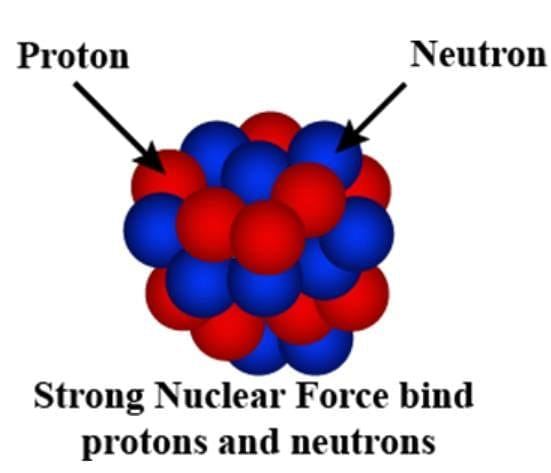This strong force will operate only when the subatomic particles are extremely close to each other, which is approximately 10-15.

So, like this, the strongest fundamental force is nature keeps nature balanced by keeping the matter in a stable form.

Additional Information: In nature, there are 4 fundamental forces. Every fundamental force plays vital importance to drive nature smoothly and knowingly or unknowingly we experience them in everyday life. A force is weak or strong which depends on the type of force it is having fundamentally.
The 4 fundamental forces are:
1. Gravitational force
2. The weak force or the weak nuclear force
3. Strong nuclear force or the strong nuclear interaction
4. Electromagnetic force

Test: Physical World - 1 - Question 3

### Which force operates among the heavier elementary particles?

Detailed Solution for Test: Physical World - 1 - Question 3

The correct answer is Strong Nuclear Force

• In post-medieval times, when atoms were being discovered, atoms were suggested to be the elementary particles of nature.
• But as science advanced, the elementary particles changed and protons, electrons and neutrons were now said to be the elementary particles.
• But, this wasn’t the end of it. According to modern science observation in Particle Physics, the most fundamental particles are finally found to be “Quarks”.
• These are said to constitute even the nucleons.
• Now, there are six different types of quarks. These are: up, down, charm, strange, top and bottom. Out of the six different types of quarks, up and down quarks have the lowest mass.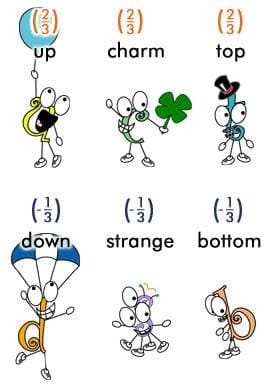• The heavier quarks interact among themselves by the strong nuclear forces.
• This is the interaction force that brings these particles together to form nucleons.

Hence, the force that operates among the heavier elementary particles is the strong nuclear force.
Note: The heavier elementary particles are relatively less stable than the lighter elementary particles, that is, the up and down quarks. So, the heavier particles rapidly transform into up and down quarks through a process of particle decay, that is, the transformation from a greater state of mass to a lower state of mass.

Test: Physical World - 1 - Question 4

Electromagnetic force is stronger than gravitational force by a factor of:

Detailed Solution for Test: Physical World - 1 - Question 4

The electromagnetic force is stronger than the gravitational force by a factor of 1036. The complete explanation of the answer is given below:

The gravitational force is directly proportional to the mass of both objects. The electrostatic force is directly proportional to the charge of the objects.

Mathematically,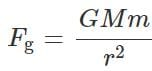and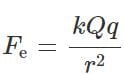• For objects on the atomic scale, for example, electrons, they have little mass, but a relatively large charge. Therefore, electromagnetic forces dominate
• For objects on the macroscopic scale such as stars, they have an overall little net charge in comparison to their huge mass. Therefore, gravitational forces dominate.
• Now, Calculate gravitational force using Newton’s law of universal gravitation and electrostatic force using Coulomb’s law given the mass and charge of the electron.
• The ratio of the resulting gravitational and electrostatic forces, Fg: Fe is on the order of 1: 1036, showing the electrostatic force is about 1036 times greater than the gravitational force.

Hence, The electromagnetic force is stronger than the gravitational force by a factor of 1036

Test: Physical World - 1 - Question 5

Detailed Solution for Test: Physical World - 1 - Question 5
• Jagadish Chandra Bose discovered the ultra-short waves. He attended Cambridge after studying physics at Calcutta University.
• He was the first to demonstrate radio communication with millimetre wavelengths, which fall in the 30GHz to 300GHz spectrum.
• However, Radio waves were first predicted by James Clerk Maxwell and Heinrich Hertz was the first to generate radio waves under experimental conditions.
Test: Physical World - 1 - Question 6

The wave nature of electrons is used in which of the following devices?

Detailed Solution for Test: Physical World - 1 - Question 6

The Correct answer is Electron Microscope.

• The electron microscope uses the wave nature of electrons for its functioning.
• It uses a beam of accelerated electrons as a source of illumination.

Electron Microscope: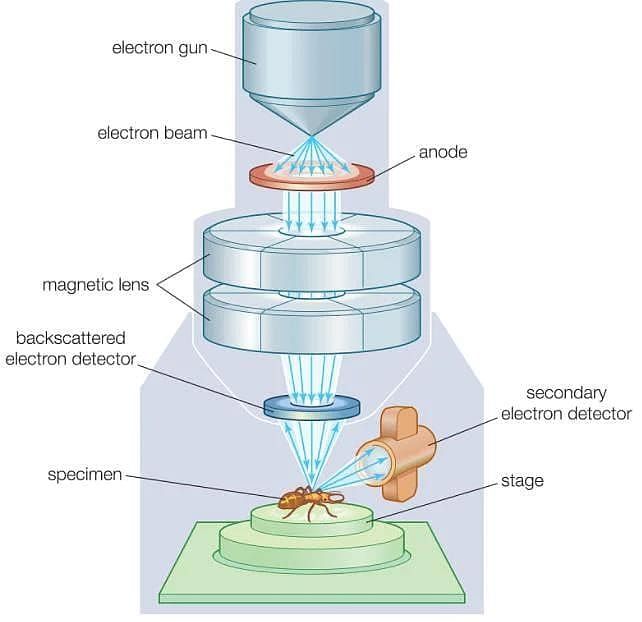Whereas,

• In a photocell, light waves are used.
• tokamak is a device that uses a powerful magnetic field to confine plasma in the shape of a torus.
• The magnetic spectrum made up of gamma rays, x-ray, ultraviolet rays, visible light, infrared, microwave and radio rays is used in an aeroplane
Test: Physical World - 1 - Question 7

According to Einstein’s theory, mass and energy are related as:

Detailed Solution for Test: Physical World - 1 - Question 7
• Einstein's equation E = mc2 shows that energy and mass are interchangeable.
• Mass-energy equivalence implies that every object possesses small energy even in a stationary position.
• But a stationary object does not have kinetic energy and it possesses potential energy and probable chemical and thermal energy.
• The sum of all these energies is smaller than the product of the mass of the object and the square of the speed of light according to the field of applied mechanics.

Hence, Mass-energy equivalence means mass and energy are the same and can be converted into each other. Einstein related the mass and energy accurately using his theory of relativity. The equation is known as Einstein’s mass-energy equation and it is given below:

E=mc2
Where

• E = equivalent kinetic energy of the object,
• m = mass of the object (Kg) and

• c = speed of light (approximately = 3×108ms-1)

Test: Physical World - 1 - Question 8

On which laws, is the principle of rocket propulsion based?

Detailed Solution for Test: Physical World - 1 - Question 8
• Propulsion is the act of moving or pushing an object forward. A propulsion system is an engine that produces thrust to push an object, such as an airplane or rocket, forward.
• The propulsion of all rockets is explained by the same physical principle: Newton’s third law of motion. Since Newton's third law of motion is not given in the options, the correct answer is None of these.
• It states that all forces exist in pairs: if one object A exerts a force FA on a second object B, then B simultaneously exerts a force FB on A, and the two forces are equal and opposite: FA = −FB.
• A rocket’s acceleration depends on three major factors: the exhaust velocity, the rate the exhaust is ejected, and the mass of the rocket.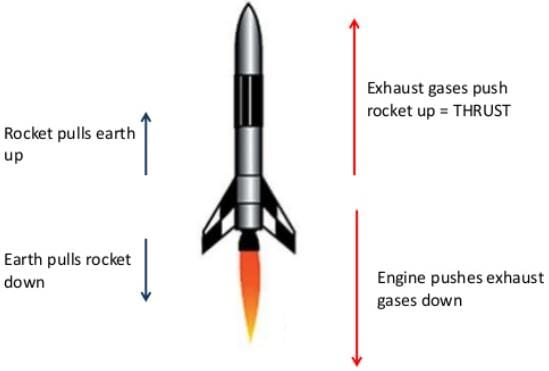• To achieve the high speeds needed to hop continents, obtain orbit, or escape Earth’s gravity altogether, the mass of the rocket other than fuel must be as small as possible.
Test: Physical World - 1 - Question 9

The phenomenon of Photoelectric effect is used in:

Detailed Solution for Test: Physical World - 1 - Question 9
• A photocell is a practical application of the phenomenon of the photoelectric effect
• When the light of frequency greater than the threshold frequency of the cathode material falls on the cathode, photoelectrons are emitted.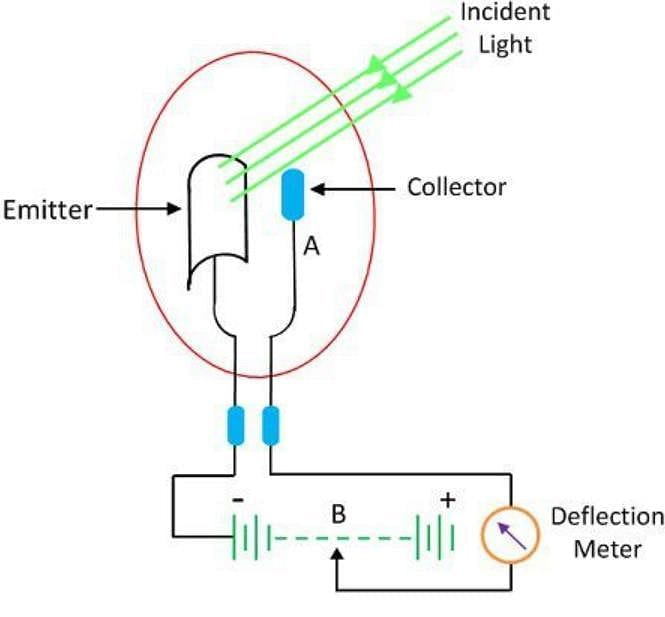• These electrons are collected by the anode and an electric current starts flowing in the external circuit.
• The current increases with the increase in the intensity of light. The current would stop, if the light does not fall on the cathode.
Test: Physical World - 1 - Question 10

Scientific method involves

Detailed Solution for Test: Physical World - 1 - Question 10
• The scientific method is a series of steps followed by scientific investigators to answer specific questions about the natural world.
• It involves making observations, formulating a hypothesis, and conducting scientific experiments.
• Scientific inquiry starts with an observation followed by the formulation of a question about what has been observed.
• The steps of the scientific method are as follows: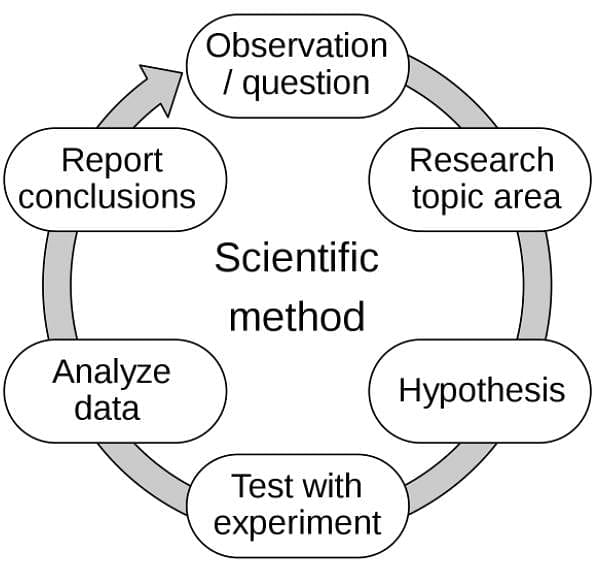## Physics Class 11

127 videos|464 docs|210 tests
 Use Code STAYHOME200 and get INR 200 additional OFF Use Coupon Code
Information about Test: Physical World - 1 Page
In this test you can find the Exam questions for Test: Physical World - 1 solved & explained in the simplest way possible. Besides giving Questions and answers for Test: Physical World - 1, EduRev gives you an ample number of Online tests for practice

## Physics Class 11

127 videos|464 docs|210 tests

### How to Prepare for NEET

Read our guide to prepare for NEET which is created by Toppers & the best Teachers